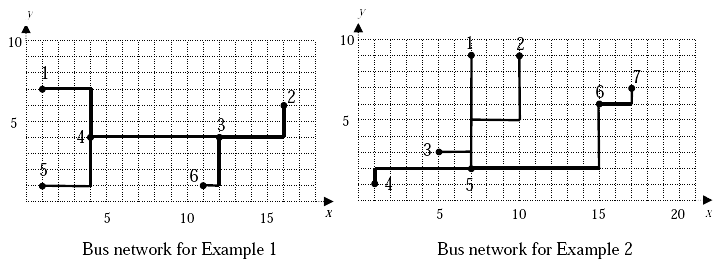## Bus Terminals

Yong-In city plans to build a bus network with N bus stops. Each bus stop is at a street corner. Yong-In is a modern city, so its map is a grid of square blocks of equal size. Two of these bus stops are to be selected as hubs H1 and H2. The hubs will be connected to each other by a direct bus line and each of the remaining N - 2 bus stops will be connected directly to either H1 or H2 (but not to both), but not to any other bus stop.

The distance between any two bus stops is the length of the shortest possible route following the streets. That is, if a bus stop is represented as (x, y) with x-coordinate x and y-coordinate y, then the distance between two bus stops (x1, y1) and (x2, y2) is |x1-x2|+|y1-y2|. If bus stops A and B are connected to the same hub H1, then the length of the route from A to B is the sum of the distances from A to H1 and from H1 to B. If bus stops A and B are connected to different hubs, e.g., A to H1 and B to H2, then the length of the route from A to B is the sum of the distances from A to H1, from H1 to H2, and from H2 to B.

The planning authority of Yong-In city would like to make sure that every citizen can reach every point within the city as quickly as possible. Therefore, city planners want to choose two bus stops to be hubs in such a way that in the resulting bus network the length of the longest route between any two bus stops is as short as possible.

One choice P of two hubs and assignments of bus stops to those hubs is better than another choice Q if the length of the longest bus route is shorter in P than in Q. Your task is to write a program to compute the length of this longest route for the best choice P.

### Input

Your program is to read from standard input. The first line contains one positive integer N (2 ≤ N ≤ 500), the number of bus stops. Each of the remaining N lines contains the x-coordinate followed by the y-coordinate of a bus stop. The x- and y-coordinates are positive integers ≤ 5000. No two bus stops are at the same location.

### Output

Your program is to write to standard output. The output contains one line with a single positive integer, the minimum length of the longest bus route for the input.

```6
1 7
16 6
12 4
4 4
1 1
11 1
```

```20
```

```7
7 9
10 9
5 3
1 1
7 2
15 6
17 7
```

### Sample Output 2

```25
```

The following figures show the bus networks for the inputs given above. If in Example 1 bus stops 3 and 4 are selected as hubs then the longest route is either between bus stops 2 and 5 or between bus stops 2 and 1. There is no better choice for the hubs, and the answer is 20.

For the bus network in Example 2, if bus stops 5 and 6 are selected as hubs then the longest route is obtained between bus stops 2 and 7. There is no better choice for the hubs, and the answer is 25.Point Value: 30 (partial)
Time Limit: 2.00s
Memory Limit: 32M